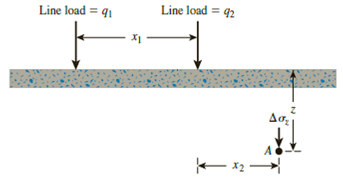Chapter 10, Problem 10.8PPrinciples of Geotechnical Enginee...

9th Edition
Braja M. Das + 1 other
ISBN: 9781305970939

Solutions

Chapter
SectionPrinciples of Geotechnical Enginee...

9th Edition
Braja M. Das + 1 other
ISBN: 9781305970939
Textbook Problem

Refer to Figure 10.41. Determine the vertical stress increase, Δσz, at point A with the following values: q1 = 110 kN/m, q2 = 440 kN/m, x1 = 6 m, x2 = 3 m, and z = 4 m.Figure 10.41

To determine

Calculate the vertical stress increase (Δσz) at point A.

Explanation

Given information:

The line load (q1) is 110kN/m.

The line load (q2) is 440kN/m.

The horizontal distance between two line loads (x1) is 6m.

The horizontal distance between line load q2 to point A (x2) is 3m.

The depth of the stress from ground surface (z) is 4m.

Calculation:

Calculate the vertical stress increase at point A (Δσz(1)) due to line load q1 by using the principles of the theory of elasticity as follows.

Δσz(1)=2q1z3π[(x1+x2)2+z2]2

Substitute 110kN/m for q1, 4m for z, 6m for x1, and 3m for x2.

Δσz(1)=2×110×43π[(6+3)2+42]2=14,080π×9,409=0.48kN/m2

Calculate the vertical stress increase at point A (Δσz(2)) due to line load q2 by using the principles of the theory of elasticity as follows

Still sussing out bartleby?

Check out a sample textbook solution.

See a sample solution

The Solution to Your Study Problems

Bartleby provides explanations to thousands of textbook problems written by our experts, many with advanced degrees!

Get Started

What is an ATC?

Precision Machining Technology (MindTap Course List)

What is a Tier I ERP software vendor?

Fundamentals of Information Systems

What is a phishing scam?

Enhanced Discovering Computers 2017 (Shelly Cashman Series) (MindTap Course List)

What emergency steps should be taken to treat burns?

Welding: Principles and Applications (MindTap Course List)